# Cube wall

The surface of the first cube wall is 64 m2. The second cube area is 40% of the surface of the first cube. Determine the length of the edge of the second cube (x).

x =  2.0656 m

### Step-by-step explanation: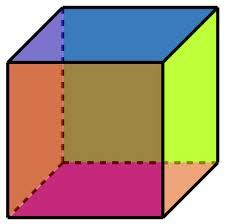Did you find an error or inaccuracy? Feel free to write us. Thank you!Tips to related online calculators

## Related math problems and questions:

• The cubeThe cube has a surface area of 216 dm2. Calculate: a) the content of one wall, b) edge length, c) cube volume.
• Cube 8The surface of the cube is 0.54 m2. Calculate the length of the cube edge.
• Cube wallsFind the volume and surface area of the cube if the area of one wall is 40cm2.
• Extending square gardenMrs. Petrová's garden had the shape of a square with a side length of 15 m. After its enlargement by 64 m2 (square), it had the shape of a square again. How many meters has the length of each side of the garden been extended?
• Area to volumeIf the surface area of a cube is 486, find its volume.
• TerezaThe cube has an area of base 256 mm2. Calculate the edge length, volume, and area of its surface.
• Length of the edgeFind the length of the edge of a cube with a cm2 surface and a volume in cm3 expressed by the same number.
• Cuboid edges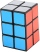The lengths of the cuboid edges are in the ratio 2: 3: 4. Find their length if you know that the surface of the cuboid is 468 m2.
• Volume and areaWhat is the volume of a cube which has an area of 361 cm2?
• Cube 5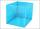The surface of the cube is 15.36 dm2. How will change the surface area of this cube if the length of the edges is reduced by 2 cm?
• Cube edgeDetermine the edges of the cube when the surface is equal to 37.5 cm square.
• Surface area 2Calculate how many % reduce the surface area of the cube is we reduced length of each edge by 10%.
• The ratio 4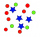The ratio of two number is 5:4 if 40% of the first number is 12, what will be 50% of the second number?
• The cubeThe cube has a surface area of 486 m ^ 2. Calculate its volume.
• Cube surface and volumeFind the surface of the cube with a volume of 27 dm3.
• The cube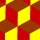The cube has an edge of 12 dm. The second cube has an edge exactly 20% longer. How many % is more water in the second cube than in the first cube, if the first cube is full to 3/4 and the second to 3/8?
• Cube wallsFind the volume and the surface area of the cube if the area of one of its walls is 40 cm2.